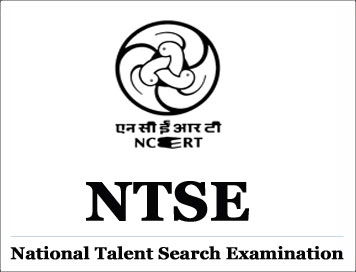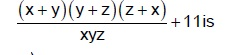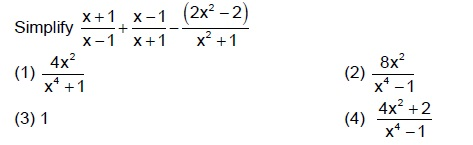# NTSE Sample Questions 2016-17 : Mental Ability (Delhi Zone)

Disclaimer: This website is NOT associated with CBSE, for official website of CBSE visit - www.cbse.gov.in

### NTSE Sample Questions 2016-17 : Mental Ability (Delhi Zone)

1. If x + y + z = 0, then value of(1) x + 11 (2) y + 11
(3) z + 11 (4) 10

2. If sinA + cosA = √ 2 sin(900 - A) then value of (√2+1)tan A will be
(1) 1 (2) 0
(3) 2 (4) 2

3. If the point (K, 2) is equidistant from the point (5, –2) and (1, –2) then value of K2 + 7 will be

(1) 10 (2) 9
(3) 12 (4) 16

4. if each side of a cube is increased by 40%, then how much percent its total surface area will be increased.
(1) 76 (2) 80
(3) 96 (4) 85

5. If sum of squares of zeros of a quadratic polynomial g(y) = y2 - 6y + p is 10. What will be the value of p.
(1) 13 (2) 12
(3) 11 (4) 10

6. A train cross a pole in 12 seconds. If the speed of the train is 54 km/hr then length of train will be
(1) 648 meter (2) 150 meter
(3) 180 meter (4) 100 meter

7. If the sum of the digits of a two digit number is 9 and the difference between the number and that formed by reversing the digits is 45 then number is
(1) 81 (2) 72
(3) 45 (4) 54

8. How many numbers between 10 and 90 are divisible by 8 completely
(1) 12 (2) 10
(3) 11 (4) 8

9.Value of x will be
(1) 14/19 (2) 17/19
(3) 15/19 (4) 41/19

10.11. Vinod has some cows and some hens in his shed. The total number of legs is 92 and total number of heads is 29. Then the number of hens in his shed is
(1) 14 (2) 12
(3) 17 (4) 21

12. Parth can row 16km downstream and 8km upstream in 6 hours. He can row 6km upstream and 24 km downstream in 6 hours. Find the speed of Parth in still water
(1) 5km/hr (2) 3km/hr
(3) 6km/hr (4) 8km/hr